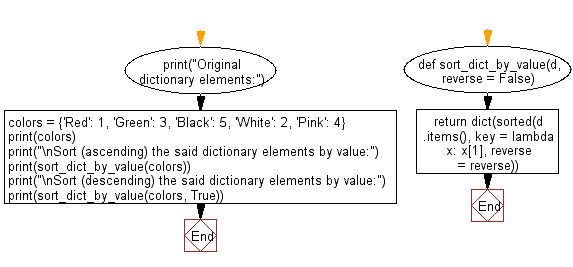﻿ Python: Sort (ascending and descending) a dictionary by value - w3resource# Python: Sort (ascending and descending) a dictionary by value

## Python dictionary: Exercise-1 with Solution

Write a Python program to sort (ascending and descending) a dictionary by value.

Sample Solution-1:

Python Code:

``````import operator
d = {1: 2, 3: 4, 4: 3, 2: 1, 0: 0}
print('Original dictionary : ',d)
sorted_d = sorted(d.items(), key=operator.itemgetter(1))
print('Dictionary in ascending order by value : ',sorted_d)
sorted_d = dict( sorted(d.items(), key=operator.itemgetter(1),reverse=True))
print('Dictionary in descending order by value : ',sorted_d)
```
```

Sample Output:

```Original dictionary :  {1: 2, 3: 4, 4: 3, 2: 1, 0: 0}
Dictionary in ascending order by value :  [(0, 0), (2, 1), (1, 2), (4, 3), (3, 4)]
Dictionary in descending order by value :  {3: 4, 4: 3, 1: 2, 2: 1, 0: 0}
```

## Visualize Python code execution:

The following tool visualize what the computer is doing step-by-step as it executes the said program:

Sample Solution-2:

Note: Dictionary values must be of the same type.

• Use dict.items() to get a list of tuple pairs from d and sort it using a lambda function and sorted().
• Use dict() to convert the sorted list back to a dictionary.
• Use the reverse parameter in sorted() to sort the dictionary in reverse order, based on the second argument.

Python Code:

``````def sort_dict_by_value(d, reverse = False):
return dict(sorted(d.items(), key = lambda x: x, reverse = reverse))
print("Original dictionary elements:")
colors = {'Red': 1, 'Green': 3, 'Black': 5, 'White': 2, 'Pink': 4}
print(colors)
print("\nSort (ascending) the said dictionary elements by value:")
print(sort_dict_by_value(colors))
print("\nSort (descending) the said dictionary elements by value:")
print(sort_dict_by_value(colors, True))
```
```

Sample Output:

```Original dictionary elements:
{'Red': 1, 'Green': 3, 'Black': 5, 'White': 2, 'Pink': 4}

Sort (ascending) the said dictionary elements by value:
{'Red': 1, 'White': 2, 'Green': 3, 'Pink': 4, 'Black': 5}

Sort (descending) the said dictionary elements by value:
{'Black': 5, 'Pink': 4, 'Green': 3, 'White': 2, 'Red': 1}
```

Flowchart:## Visualize Python code execution:

The following tool visualize what the computer is doing step-by-step as it executes the said program:

Python Code Editor:

Have another way to solve this solution? Contribute your code (and comments) through Disqus.

What is the difficulty level of this exercise?

Test your Programming skills with w3resource's quiz.

﻿

## Python: Tips of the Day

Decapitalizes the first letter of a string:

Example:

```def tips_decapitalize(s, upper_rest=False):
return s[:1].lower() + (s[1:].upper() if upper_rest else s[1:])
print(tips_decapitalize('PythonTips'))
print(tips_decapitalize('PythonTips', True))
```

Output:

```pythonTips
pYTHONTIPS
```